# Predict the initial and final products for the following reaction involving 2-pentyne. Hint: the products are isomers...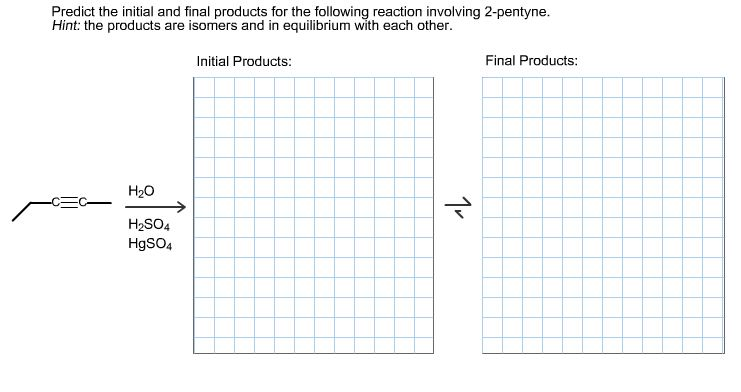Predict the initial and final products for the following reaction involving 2-pentyne. Hint: the products are isomers and in equilibrium with each other.

Concepts and reason

Hydration is the process of adding water to unsaturated compounds like alkenes or alkynes to form alcohol. Addition of water to an alkyne can be done by using a strong acid in a mercury catalyst, which then forms enols that undergo tautomerism to form ketone. Mercury is used to avoid the formation of less stable carbocation from an alkyne.

Fundamentals

The mechanism of mercury-catalyzed addition of water to an alkyne:-

Step1: Formation of intermediate called mercurinium ion.

Step2: Ring-opening reaction by the nucleophilic attack of water to form an oxonium ion.

Step3: Formation of enol through deprotonation and demercuration.

Step4: Enol to keto conversion - enol acts as a nucleophile; it reacts with an electrophile at α-carbon, which has an acidic oxygen atom $\left( {{\rm{O - H}}} \right)$ . The hydrogen present at the acidic oxygen atom acts as a bond donor and acceptor.1.2.1.2.1.2.Ans:#### Earn Coin

Coins can be redeemed for fabulous gifts.

Similar Homework Help Questions
• ### Predict the initial and final products for the following reaction involving 2-pentyne Hint: the products are isomers a...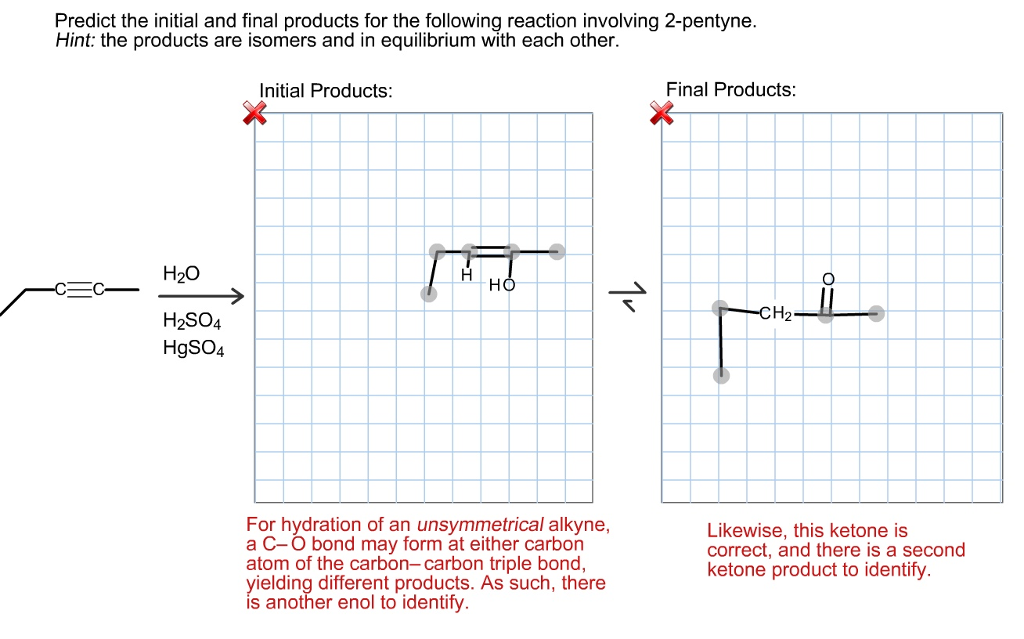Predict the initial and final products for the following reaction involving 2-pentyne Hint: the products are isomers and in equilibrium with each other. Initial Products Final Products H2SO4 HgSO4 For hydration of an unsymmetrical alkyne, a C-O bond may form at either carbon atom of the carbon-carbon triple bond, yielding different products. As such, there is another enol to identify Likewise, this ketone is correct, and there is a second ketone product to identify

• ### (9-23) Predict the initial and final products for the following reaction involving 2-pentyne....Predict the initial and final products for the following reaction involving 2-pentyne. Hint: the products are isomers and in equilibrium with each other.

• ### Predict the initial and isolated products for the following reaction. Hint: the products are isomers and...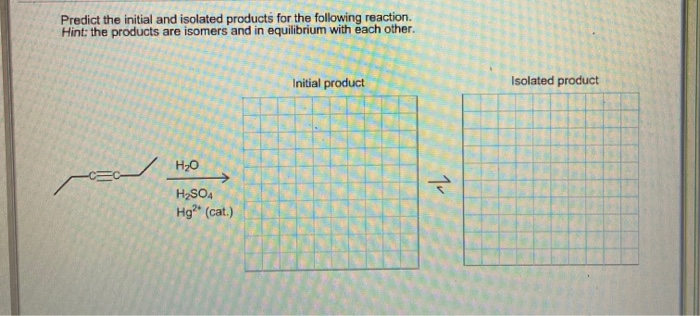Predict the initial and isolated products for the following reaction. Hint: the products are isomers and in equilibrium with each other. Initial produ Isolated product H2O H2SO Hg?" (cat.)

• ### Predict the organic products from reaction of 2-pentyne with Li in NH3- • Consider EZ stereochemistry...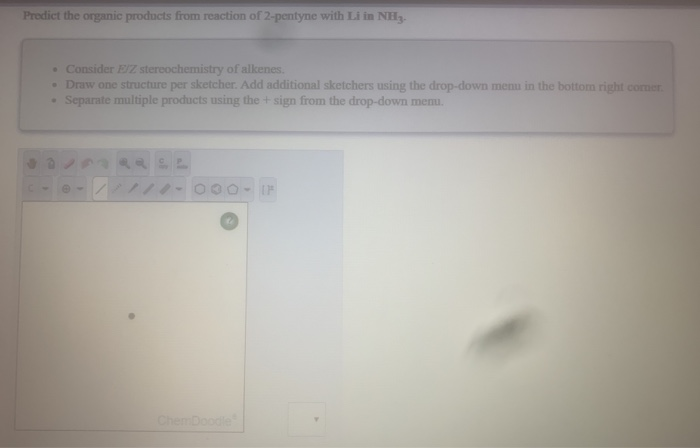Predict the organic products from reaction of 2-pentyne with Li in NH3- • Consider EZ stereochemistry of alkenes. • Draw one structure per sketcher. Add additional sketchers using the drop-down menu in the bottom right comer • Separate multiple products using the sign from the drop-down menu. - -/ -O0O-

• ### (a) (5 points) Predict the products for the following acid-base reaction. Then, predict the direction of...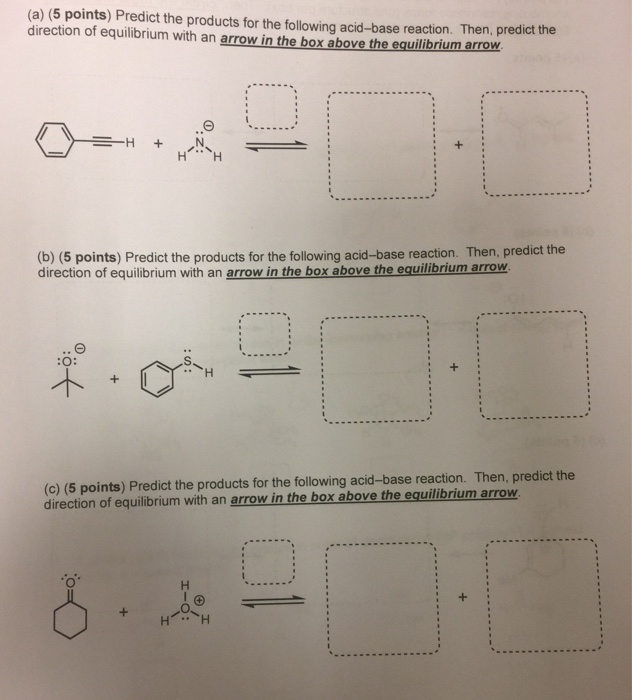(a) (5 points) Predict the products for the following acid-base reaction. Then, predict the direction of equilibrium with an arrow in the box above the equilibrium arrow (b) (5 points) Predict the products for the following acid-base reaction. Then, predict the direction of equilibrium with an arrow in the box above the equilibrium arrow (c) (5 points) Predict the products for the following acid-base reaction. Then, predict the direction of equilibrium with an arrow in the box above the equilibrium...

• ### Write out the products of the following reactions. Then, for each reaction, predict whether the products...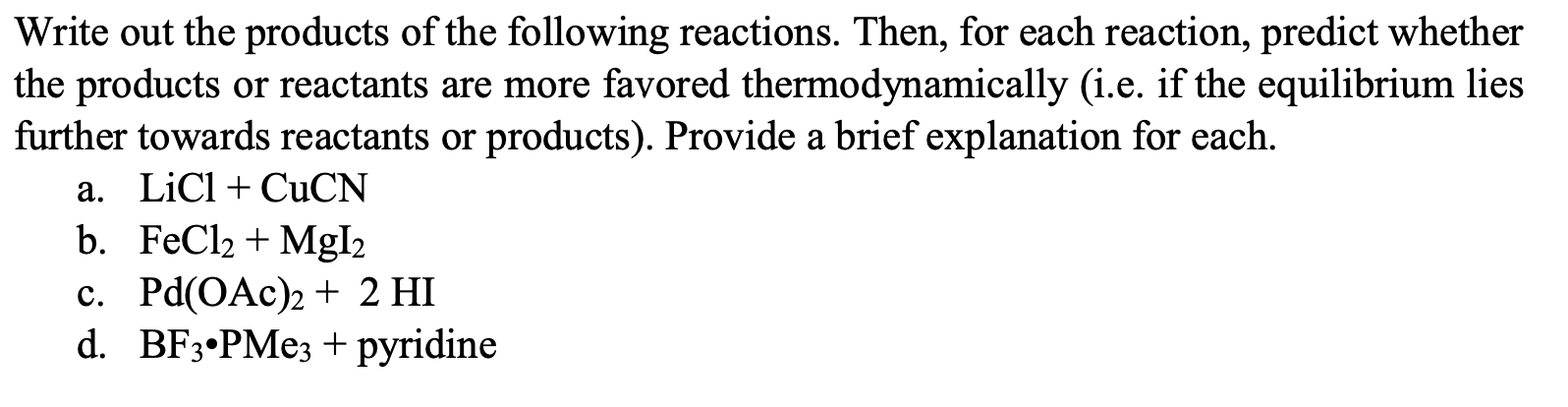Write out the products of the following reactions. Then, for each reaction, predict whether the products or reactants are more favored thermodynamically (i.e. if the equilibrium lies further towards reactants or products). Provide a brief explanation for each. a. LiCl + CuCN b. FeCl2 + MgI2 c. Pd(OAc)2 + 2 HI d. BF3•PMe3 + pyridine

• ### What is the difference between the following two isomers when treated with H2SO4? Give the final products in each ca...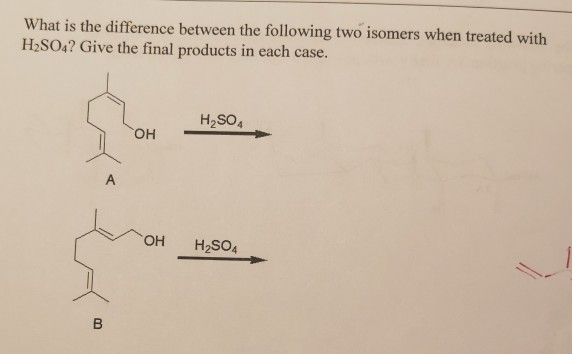What is the difference between the following two isomers when treated with H2SO4? Give the final products in each case. H2SO4 OH A OH H2SO4 What is the difference between the following two isomers when treated with H2SO4? Give the final products in each case. H2SO4 OH A OH H2SO4

• ### Question #2 Predict the products of the following reactions involving amines NH 1.) LiAIH, THF 2.)...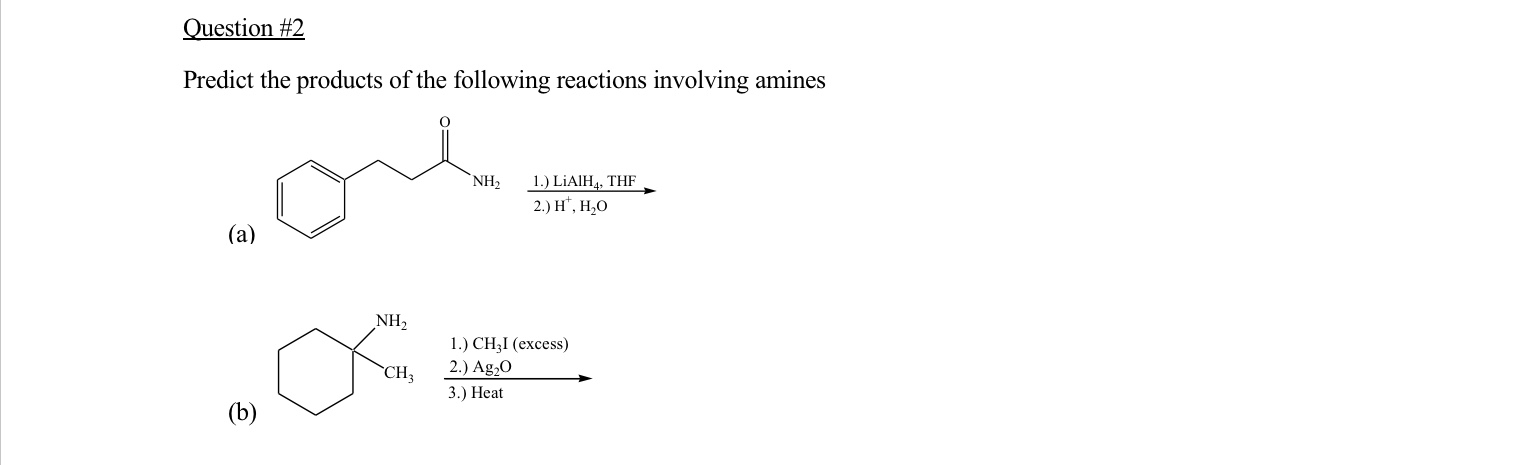Question #2 Predict the products of the following reactions involving amines NH 1.) LiAIH, THF 2.) H, H2O NH CH 1.) CHI (excess) 2.) Ag20 3.) Heat (b)

• ### Predict the products of the following reaction 1) Predict the product(s) of the following reaction. HCI...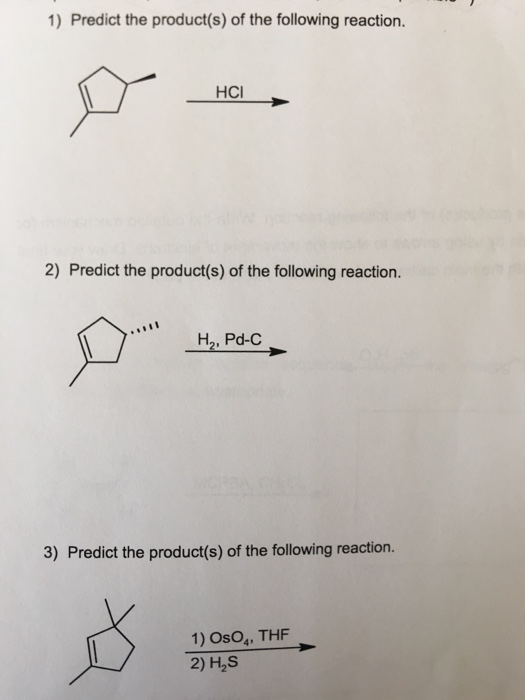Predict the products of the following reaction 1) Predict the product(s) of the following reaction. HCI 2) Predict the product(s) of the following reaction. H2, Pd-C 3) Predict the product(s) of the following reaction. 1) OsO4, THF 2) H2S

• ### The reaction of 2-pentyne with Na/NH3 gives the following product: (1) cis-2-pentene (2) trans-2-pentene (3) 1-pentyne...

The reaction of 2-pentyne with Na/NH3 gives the following product: (1) cis-2-pentene (2) trans-2-pentene (3) 1-pentyne (4) pentane

Free Homework Help App